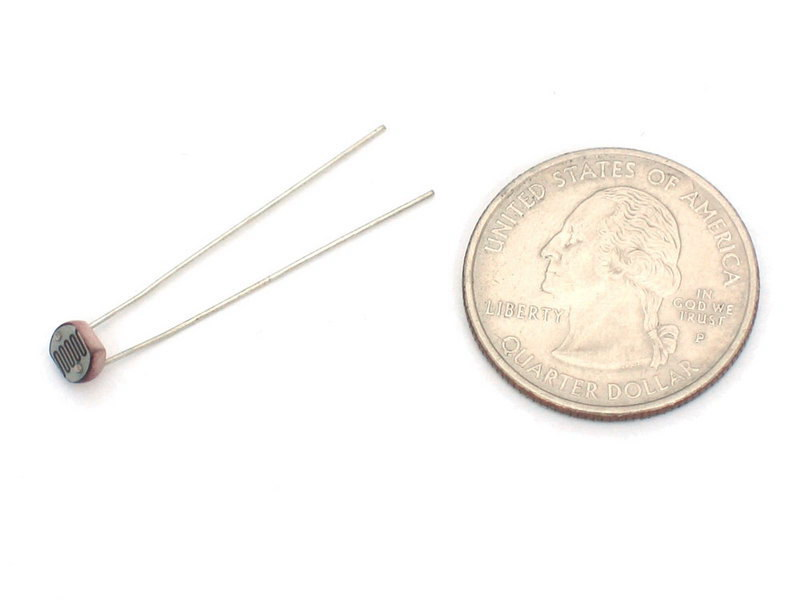Posted by: Chao Category: Sensing and Actuating Comments: One Comment

## Photocells Tutorial

1. Priciple

Photocells are sensors that allow you to detect light. They are small, inexpensive, low-power, easy to use and don’t wear out. For that reason they often appear in toys, gadgets and appliances. They are often referred to as CdS cells (they are made of Cadmium-Sulfide), light-dependent resistors (LDR), and photoresistors.

Photocells are basically a resistor that changes its resistive value (in ohms Ω) depending on how much light is shining onto the squiggly face. They are very low cost, easy to get in many sizes and specifications, but are very inaccurate. Each photocell sensor will act a little differently than the other, even if they are from the same batch. The variations can be really large, 50% or higher! For this reason, they shouldn’t be used to try to determine precise light levels in lux or millicandela. Instead, you can expect to only be able to determine basic light changes.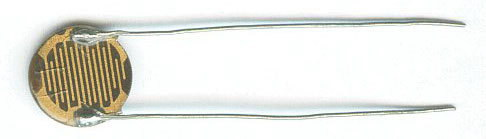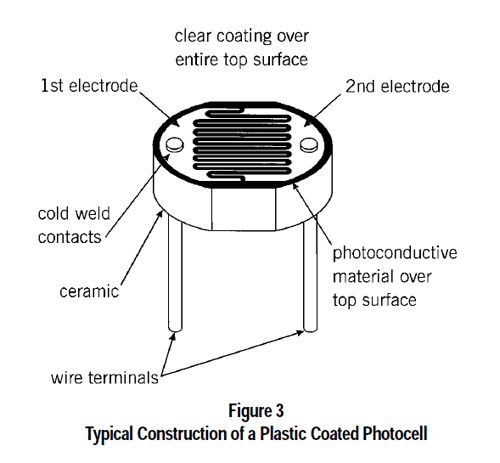2. How to select a good sensitive photocells?

There are three important features we believe you can check if this is a good one, from the datasheet, you can use “sensitivity” parameter directly to see,however, not all the phtocell has a full and comprehensive datasheet, if you only see Dark Resistance (Rd), which is corresponding to Dark current and Illuminated Resistance (Ri) which is correscponding to Illuminated current, it’s better to choose the Rd with bigger value and Ri with small value.

The sensitivity is depends on the light current, which equal to Illuminated current –  Dark current. so the Rd bigger the better, and Ri smaller the better.

3. How to measure the value used by voltage diverter?

A voltage divider is just two resistors in series connected between a voltage supply and ground.  If R1 is connected to the voltage supply and R2 is connected to ground then the voltage at the junction between the two resistors is: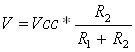If R1 is the photoresistor, the voltage will increase with increasing light intensity.  If R2 is the photoresistor, the voltage will decrease with increasing light intensity.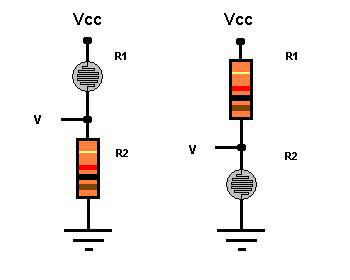4. Hook it on Arduino

The LDR changes its resistance with light so we can measure that change using one of the Arduino’s analog pins. But to do that we need a fixed resistor (not changing) that we can use for that comparison (We are using a 10K resistor). This is called a voltage divider and divides the 5v between the LDR and the resistor. Then we measure how much voltage is on the LDR using the analog read on your arduino, and we have our reading. The amount of that 5V that each part gets is proportional to its resistance.

With the arduino analogRead, at 5V (its max) it would read 1023, and at 0v it read 0.

So if the the LDR and the resistor have the same resistance, the 5V is split evenly (2.5V), to each part. (analogRead of 512)

But if the LDR is hit with a ton of light and is reading only 1K of resistance, the 10K resistor is going to soak up 10 times as much of that 5V. So the LDR would only get .45V (analogRead of 92).

And if it is in a dark room, the LDR may be 40K or resistance, so the LDR will soak up 4 times as much of that 5V as the 10K resistor. So the LDR would get 4V (analogRead of 818).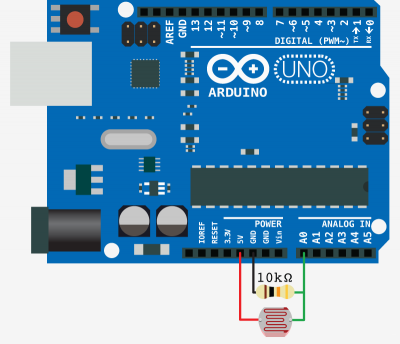Code:
[c]
int LDR_Pin = A0; //analog pin 0

void setup(){
Serial.begin(9600);
}

void loop(){

delay(250); //just here to slow down the output for easier reading
}

[/c]

## Some Basic Stats

These stats are for the photocell in the Electrodragon shop which is very much like the PDV-P8001 . Nearly all photocells will have slightly different specifications, although they all pretty much work the same. If there’s a datasheet, you’ll want to refer to it

• Size: Round, 5mm (0.2″) diameter. (Other photocells can get up to 12mm/0.4″ diameter!)
• Price: \$0.7 for 5pcs 5800B version, and \$0.8 for 20pcs 5528 version
• Resistance range: 200KΩ (dark) to 10KΩ (10 lux brightness)
• Sensitivity range: CdS cells respond to light between 400nm (violet) and 600nm (orange) wavelengths, peaking at about 520nm (green).
• Power supply: pretty much anything up to 100V, uses less than 1mA of current on average (depends on power supply voltage)
• Datasheet and another Datasheet
• Two application notes on using and selecting photocells where nearly all of these graphs are taken from